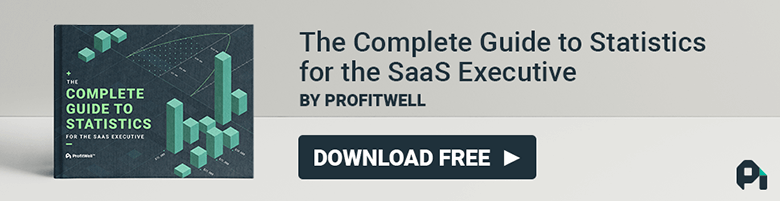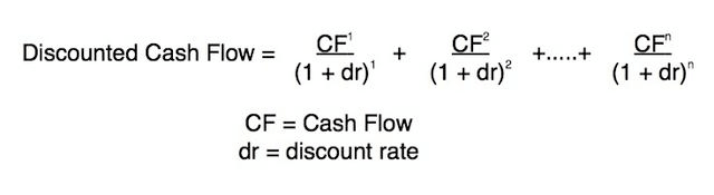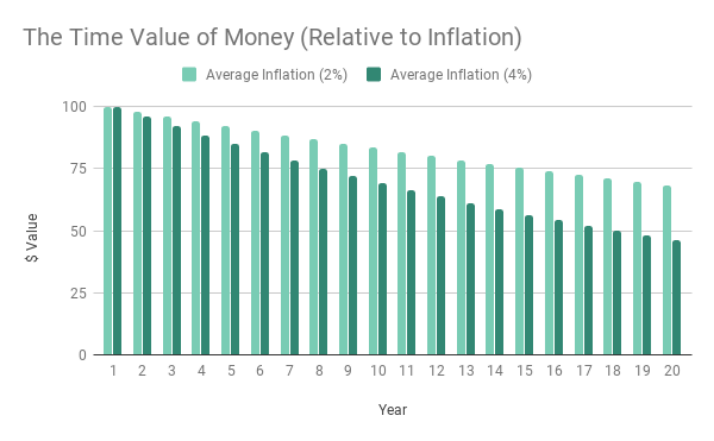# Discount Rate Formula: Calculating Discount Rate [WACC/APV]

###### Patrick Campbell Aug 16 2019

Let’s say you’re the CEO of WellProfit, a growing, Boise-based SaaS company that’s bound for the stars and thinking about getting investors. One of the first things you need to do to make your company attractive to investors is find your discount rate.

Measuring your discount rate as a business, however, can be a complex proposition. For both companies and investors, discount rate is a key metric when positioning for the future. An accurate discount rate is crucial to investing and reporting, as well as assessing the financial viability of new projects within your company.

Setting a discount rate is not always easy, and to do it precisely, you need to have a grasp of the discount rate formula. Finding your discount rate involves an array of factors that have to be taken into account, including your company’s equity, debt, and inventory. Doing it right, however, is key to understanding the future worth of your company compared to its value now and, ultimately, bridging that gap.

## What is a discount rate?

The definition of a discount rate depends the context, it's either defined as the interest rate used to calculate net present value or the interest rate charged by the Federal Reserve Bank. There are two discount rate formulas you can use to calculate discount rate, WACC (weighted average cost of capital) and APV (adjusted present value).### Interest rate used to calculate Net Present Value (NPV)

The discount rate we are primarily interested in concerns the calculation of your business’ future cash flows based on your company’s net present value, or NPV. Your discount rate expresses the change in the value of money as it is invested in your business over time.

You need to know your NPV when performing discounted cash flow (DCF) analysis, one of the most common valuation methods used by investors to gauge the value of investing in your business. If your company’s future cash flow is likely to be much higher than your present value, and your discount rate can help show this, it can be the difference between being attractive to investors and not.

### Interest rate charged by Federal Reserve Bank

The second utility of the term discount rate in business concerns the rate charged by banks and other financial institutions for short-term loans. It’s a very different matter and is not decided by the discount rate formulas we’ll be looking at today.

## NPV and DCF

As stated above, net present value (NPV) and discounted cash flow (DCF) are methods of valuation used to assess the quality of an investment opportunity, and both of them use discount rate as a key element.

### Net Present Value

NPV is the difference between the present value of a company’s cash inflows and the present value of cash outflows over a given time period. Your discount rate and the time period concerned will affect calculations of your company’s NPV.

NPV is used to measure the costs and benefits, and ultimately the profitability, of a prospective investment over time. It takes inflation and returns into account and features particularly in capital budgeting and investment planning - there’s even a specific Excel function for it. Otherwise, you can calculate it like so:The discount rate element of the NPV formula is used to account for the difference between the value-return on an investment in the future and the money to be invested in the present. Your company’s weighted average cost of capital (WACC, a discount rate formula we’ll show you how to calculate shortly) is often used as the discount rate when calculating NPV, although it is sometimes thought to be more appropriate to use a higher discount rate to adjust for risk or opportunity cost.

Some investors may wish to use a specific figure as a discount rate, depending on their projected return - for instance, if investment funds are to be used to target a specific rate of return, then this rate of return may be used as the discount rate when calculating NPV.

You, as the hypothetical CEO of WellProfit, might find yourself asked to present the net present value of a solution-building project that requires an initial investment of \$250,000. It is expected to bring in \$40,000 per month of net cash flow over a 12-month period with a target rate of return of 10%, which will act as our discount rate.

NPV = 40,000(Month 1)/1 + 0.1 + 40,000 (Month 2)/1 + 0.1 ... - 250,000

= \$230,000

This NPV is not only positive but very high; an investor is likely to go through with the investment, which is good news for WellProfit!

### Discounted Cash Flow

DCF is a method of valuation that uses the future cash flows of an investment in order to estimate its value. You can calculate it like so:As the hypothetical CEO of WellProfit, you’d first calculate your discount rate and your NPV (which, remember, is the difference between the present value of cash inflows and the present value of cash outflows over a period of time and is represented above by “CF”).

Then you can perform a DCF analysis that estimates and discounts the value of all future cash flows by cost of capital to gain a picture of their present values. If this value proves to be higher than the cost of investing, then the investment possibility is viable. Let’s say you have an investor looking to invest in a 20% stake in your company; you're growing at 14.1% per year and produce \$561,432 per year in free cash flow, giving your investor a cash return of \$112,286 per year. How much is that 20% stake worth now?

The investor will assess the amount they’ll earn this year (\$112,286), in year two (\$112,286 x 1.141 = \$128,118), and so on. We’ll change our discount rate from our previous NPV calculation. Let’s say now that the target compounded rate of return is 30% per year; we’ll use that 30% as our discount rate. Calculate the amount they earn by iterating through each year, factoring in growth.

You’ll find that, in this case, discounted cash flow goes down (from \$86,373 in year one to \$75,809 in year two, etc.) because your discount rate is higher than your current growth rate. Therefore, it’s unlikely that, at this growth rate and discount rate, an investor will look at this one as a bright investment prospect. Bad news for WellProfit.

To put it briefly, DCF is supposed to answer the question: "How much money would have to be invested currently, at a given rate of return, to yield the forecast cash flow at a given future date?" You can find out more about how DCF is calculated here and here.

## What is discount rate used for?

Discount rate is used primarily by companies and investors to position themselves for future success. For companies, that entails understanding the future value of their cash flows and ensuring development is kept within budget. For investors, the discount rate allows them to assess the viability of an investment based on that relationship of value-now to value-later.

### Accounting for the time value of money

Money, as the old saying goes, never sleeps. Owing to the rule of earning capacity, a dollar at a later point in time will not have the same value as a dollar right now. This principle is known as the “time value of money.” We can see how the value of a given sum gradually decreases over time here.As this value is changed by the accumulation of interest and general inflation, as well as by profits and discounts from investments, it’s handy to have the discount rate calculated as a roadmap of where the value of a dollar invested in your business is likely to go.

For instance, if an investor offers your company \$1 million for the promise of receiving \$7 million in five years’ time, the promise to receive that \$7 million 30 years in the future would be worth much less today from the investor’s point of view, even if they were guaranteed payback in both cases (and even though it’s still \$7 million dollars!).

Present value (PV), future value (FV), investment timeline measured out in periods (N), interest rate, and payment amount (PMT) all play a part in determining the time value of money being invested. We’ll see a number of those variables included in our discount rate formulas.

### Determining potential value / risk factor of future investments

Being able to understand the value of your future cash flows by calculating your discount rate is similarly important when it comes to evaluating both the value potential and risk factor of new developments or investments.

From your company’s side, you can only go ahead with a new project if expected revenue outweighs the costs of pursuing said opportunity. Knowing your discount rate is key to understanding the shape of your cash flow down the line and whether your new development will generate enough revenue to offset the initial expenses.

From the perspective of an investor, including your company’s discount rate in their calculations makes it easier to accurately estimate how much the project's future cash flows are worth now and the size of the present investment needed in order to make an investment profitable.

### Calculating NPV (as part of DCF analysis)

As we noted earlier, you can’t gain a full picture of your company's future cash flows without solid DCF analysis; you can't perform DCF analysis without calculating NPV; you can't calculate either without knowing your discount rate.

Without knowing your discount rate, you can’t precisely calculate the difference between the value-return on an investment in the future and the money to be invested in the present. Once you have your NPV calculated this way, you can pair it with your discount rate to get a sense of your DCF.

## How to calculate discount rate

There are two primary discount rate formulas - the weighted average cost of capital (WACC) and adjusted present value (APV). The WACC discount formula is: WACC = E/V x Ce + D/V x Cd x (1-T), and the APV discount formula is: APV = NPV + PV of the impact of financing. Let’s dive deeper into these two formulas and how they’re different below.

### Weighted Average Cost of Capital (WACC)

WACC can be used to calculate the enterprise value of a firm by considering the cost of goods available for sale against inventory, alongside common stock, preferred stock, bonds, and any other long-term debt on your company’s books.

It is comprised of a blend of the cost of equity and after-tax cost of debt and is calculated by multiplying the cost of each capital source (debt and equity) by its relevant weight and then adding the products together to determine the WACC value.

The WACC formula for discount rate is as follows:

WACC = E/V x Ce + D/V x Cd x (1-T)

Where:

• E = Value of equity
• D = Value of debt
• Ce = Cost of equity
• Cd = Cost of debt
• V = D + E
• T = Tax rate

This discount rate formula can be modified to account for periodic inventory (the cost of goods available for sale, and the units available for sale at the end of the sales period) or perpetual inventory (the average before the sale of units).

Let’s break it down, and let’s presume WellProfit has taken off and absolutely exploded, and we want to calculate WACC to get a sense of our enterprise value. Let’s say that shareholder equity (E) for the year 2030 will be \$4.2 billion and the long-term debt (D) stands at \$1.1 billion.

Our overall capital = E + D = 4.2 billion + 1.1 billion = \$5.3 billion

The equity linked cost of capital = (E/V) x Re = 4.2/5.3 x 6.6615% = 0.0524

The debt component = (D/V) x Cd x (1-T) = 1.1/5.3 x 6.5% x (1-21%) = - 0.0197

WACC = 0.0524 + -0.0197 = 3.2%

Our second discount rate formula, the adjusted present value calculation, makes use of NPV. APV analysis tends to be preferred in highly leveraged transactions; unlike a straightforward NPV valuation, it “takes into consideration the benefits of raising debts (e.g., interest tax shield)."

APV can also be useful when revealing the hidden value of seemingly less viable investment opportunities. By considering financing investment with a portion of debt, some prospects that might’ve looked unviable with NPV alone suddenly seem more attractive as investment possibilities.

This second discount rate formula is fairly simple and uses the cost of equity as the discount rate:

APV = NPV + PV of the impact of financing

Where:

• NPV = Net Present Value
• PV = Present Value

Discount rate is key to managing the relationship between an investor and a company, as well as the relationship between a company and its future self.

The health of cash flow, not just now but in the future, is fundamental to the health of your business - 82% of all startups without reliable cash flows will ultimately fold. Investing in one is a risk, and investors need to know that the value of your cash flows will hold not only now but also later.

In order to manage your own expectations for your company, and in order for investors to vet the quality of your business as an investment opportunity, you need to know how to find that discount rate. Using the right discount rate formula, setting the right rate relative to your equity, debt, inventory, and overall present value is paramount.##### By Patrick Campbell

Founder & CEO of ProfitWell, the software for helping subscription companies with their monetization and retention strategies, as well as providing free turnkey subscription financial metrics for over 20,000 companies. Prior to ProfitWell Patrick led Strategic Initiatives for Boston-based Gemvara and was an Economist at Google and the US Intelligence community.